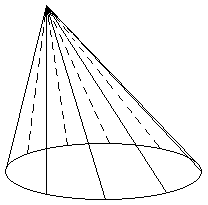# Cones

A cone is the body obtained from rotating a rectanglular triangle around one of its cathetus. The elements of the cone are:• The axis is the fixed cathetus around which the triangle turns.

• The base is the circle generated by the other cathetus.

• The generator side is the hypotenuse of the rectanglular triangle.

• The height is the distance between the vertex and the base.

Truncated Cone: It is the geometric body that results form cutting a cone with a plane parallel to the base. The section determined by the cut is the lower base.## Calculation of the generator side

• Generator of the cone:The cone is a body generated by a rectanglular triangle turning around one of its cathetus. Its height will be that cathetus, and the hypotenuse will be its generator. From the Pythagoras' theorem the generator of the cone will be equal to: $$g=\sqrt{h^2+r^2}$$$• Generator of the truncated cone:The truncated cone is a body generated by a rectangular trapeze turning around the side perpendicular to the base. This will also be its height and the other side will be the generator. We obtain the generator of the trunk of cone applying Pythagoras' theorem in the shaded triangle: $$g=\sqrt{h^2+(R-r)^2}$$$

## Classification of the cones

• The straight cone has its vertex equidistant to the circular base.• The oblique cone has the vertex non equidistant to its base.